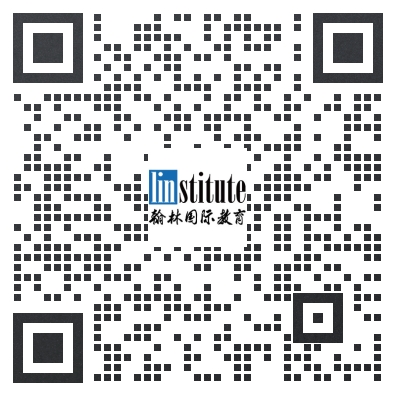# 2016 USAJMO Problems真题及答案

+答案解析请参考文末

## Day 1

### Problem 1

The isosceles triangle$\triangle ABC$, with$AB=AC$, is inscribed in the circle$\omega$. Let$P$ be a variable point on the arc$\stackrel{\frown}{BC}$ that does not contain$A$, and let$I_B$ and$I_C$ denote the incenters of triangles$\triangle ABP$ and$\triangle ACP$, respectively.

Prove that as$P$ varies, the circumcircle of triangle$\triangle PI_BI_C$ passes through a fixed point.

### Problem 2

Prove that there exists a positive integer$n < 10^6$ such that$5^n$ has six consecutive zeros in its decimal representation.

### Problem 3

Let$X_1, X_2, \ldots, X_{100}$ be a sequence of mutually distinct nonempty subsets of a set$S$. Any two sets$X_i$ and$X_{i+1}$ are disjoint and their union is not the whole set$S$, that is,$X_i\cap X_{i+1}=\emptyset$ and$X_i\cup X_{i+1}\neq S$, for all$i\in\{1, \ldots, 99\}$. Find the smallest possible number of elements in$S$.

## Day 2

### Problem 4

Find, with proof, the least integer$N$ such that if any$2016$ elements are removed from the set$\{1, 2,\dots,N\}$, one can still find$2016$ distinct numbers among the remaining elements with sum$N$.

### Problem 5

Let$\triangle ABC$ be an acute triangle, with$O$ as its circumcenter. Point$H$ is the foot of the perpendicular from$A$ to line$\overleftrightarrow{BC}$, and points$P$ and$Q$are the feet of the perpendiculars from$H$ to the lines$\overleftrightarrow{AB}$ and$\overleftrightarrow{AC}$, respectively.

Given that$$AH^2=2\cdot AO^2,$$prove that the points$O,P,$ and$Q$ are collinear.

### Problem 6

Find all functions$f:\mathbb{R}\rightarrow \mathbb{R}$ such that for all real numbers$x$ and$y$,$$(f(x)+xy)\cdot f(x-3y)+(f(y)+xy)\cdot f(3x-y)=(f(x+y))^2.$$

### 2016USAJMO真题参考答案及详解## 报名及辅导请联系